## Representation and Visualization of Data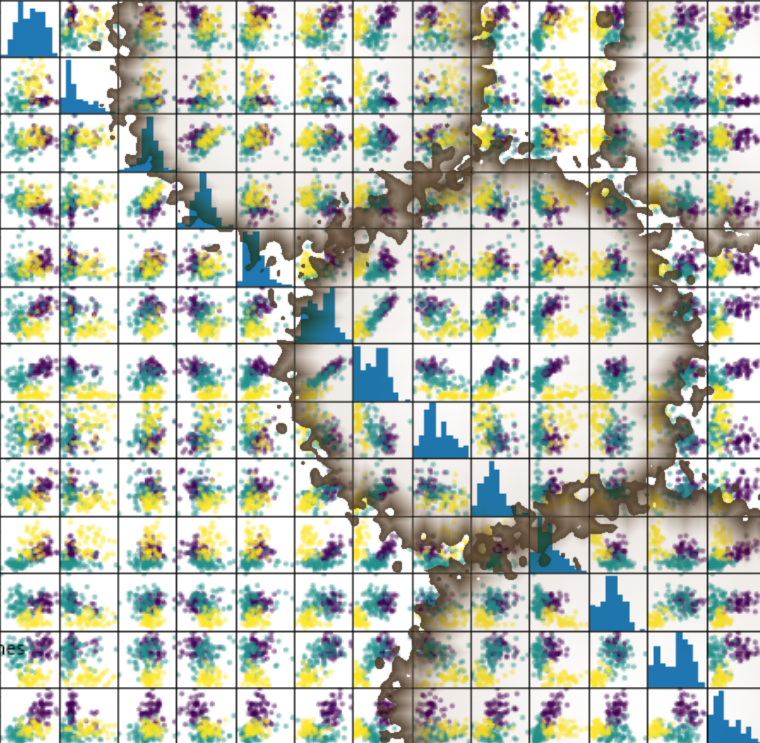At the beginning of this chapter we quoted Tom Mitchell's definition of machine learning: "Well posed Learning Problem: A computer program is said to learn from experience E with respect to some task T and some performance measure P, if its performance on T, as measured by P, improves with experience E." Data is the "raw material" for machine learning. It learns from data. In Mitchell's definition, "data" is hidden behind the terms "experience E" and "performance measure P". As mentioned earlier, we need labeled data to learn and test our algorithm.

However, it is recommended that you familiarize yourself with your data before you begin training your classifier.

Numpy offers ideal data structures to represent your data and Matplotlib offers great possibilities for visualizing your data.

• n_samples: The number of samples. A sample can be a text or a document, a picture, a sound, some measurements. They are quite often represented a row in a CSV or Excel file,

• n_features: The number of features used to describe each sample. Features are in most cases numerical values (integers, float or boolean).

## "Hello World" of Machine Learning

What was the first program you saw? I bet it might have been a program giving out "Hello World" in some programming language. Most likely I'm right. Almost every introductory book or tutorial on programming starts with such a program. It's a tradition that goes back to the 1968 book "The C Programming Language" by Brian Kernighan and Dennis Ritchie!

The likelihood that the first dataset you will see in an introductory tutorial on machine learning will be the "Iris dataset" is similarly high. The Iris dataset contains the measurements of 150 iris flowers from 3 different species:

• Iris-Setosa,
• Iris-Versicolor, and
• Iris-Virginica.

Iris SetosaIris VersicolorIris VirginicaThe iris dataset is often used for its simplicity. This dataset is contained in scikit-learn, but before we have a deeper look into the Iris dataset we will look at the other datasets available in scikit-learn.

For example, scikit-learn has a very straightforward set of data on these iris species. The data consist of the following:

• Features in the Iris dataset:

1. sepal length in cm
2. sepal width in cm
3. petal length in cm
4. petal width in cm
• Target classes to predict:

1. Iris Setosa
2. Iris Versicolour
3. Iris Virginica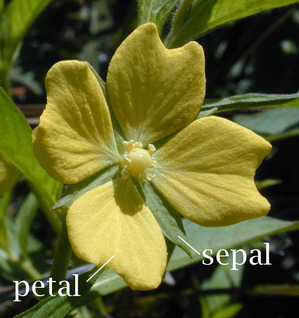(Image: "Petal-sepal". Licensed under CC BY-SA 3.0 via Wikimedia Commons - https://commons.wikimedia.org/wiki/File:Petal-sepal.jpg#/media/File:Petal-sepal.jpg)

scikit-learn embeds a copy of the iris CSV file along with a helper function to load it into numpy arrays:

from sklearn.datasets import load_iris


The resulting dataset is a Bunch object: you can see what's available using the method keys():

iris.keys()

Output: :
dict_keys(['data', 'target', 'target_names', 'DESCR', 'feature_names', 'filename'])
type(iris)

Output: :
sklearn.utils.Bunch

The features of each sample flower are stored in the data attribute of the dataset:

n_samples, n_features = iris.data.shape
print('Number of samples:', n_samples)
print('Number of features:', n_features)
# the sepal length, sepal width, petal length and petal width of the first sample (first flower)
print(iris.data)

Number of samples: 150
Number of features: 4
[5.1 3.5 1.4 0.2]


Data in scikit-learn is in most cases saved as two-dimensional Numpy arrays with the shape (n, m). Many algorithms also accept scipy.sparse matrices of the same shape.

• n: (n_samples) The number of samples: each sample is an item to process (e.g. classify). A sample can be a document, a picture, a sound, a video, an astronomical object, a row in database or CSV file, or whatever you can describe with a fixed set of quantitative traits.
• m: (n_features) The number of features or distinct traits that can be used to describe each item in a quantitative manner. Features are generally real-valued, but may be Boolean or discrete-valued in some cases.

The information about the class of each sample of our Iris dataset is stored in the target attribute of the dataset:

print(iris.target)

[0 0 0 0 0 0 0 0 0 0 0 0 0 0 0 0 0 0 0 0 0 0 0 0 0 0 0 0 0 0 0 0 0 0 0 0 0
0 0 0 0 0 0 0 0 0 0 0 0 0 1 1 1 1 1 1 1 1 1 1 1 1 1 1 1 1 1 1 1 1 1 1 1 1
1 1 1 1 1 1 1 1 1 1 1 1 1 1 1 1 1 1 1 1 1 1 1 1 1 1 2 2 2 2 2 2 2 2 2 2 2
2 2 2 2 2 2 2 2 2 2 2 2 2 2 2 2 2 2 2 2 2 2 2 2 2 2 2 2 2 2 2 2 2 2 2 2 2
2 2]


Beside of the shape of the data, we can also check the shape of the labels, i.e. the target.shape:

Each flower sample is one row in the data array, and the columns (features) represent the flower measurements in centimeters. For instance, we can represent this Iris dataset, consisting of 150 samples and 4 features, a 2-dimensional array or matrix $\mathbb{R}^{150 \times 4}$ in the following format:

$$\mathbf{X} = \begin{bmatrix} x_{1}^{(1)} & x_{2}^{(1)} & x_{3}^{(1)} & x_{4}^{(1)} \\ x_{1}^{(2)} & x_{2}^{(2)} & x_{3}^{(2)} & x_{4}^{(2)} \\ \vdots & \vdots & \vdots & \vdots \\ x_{1}^{(150)} & x_{2}^{(150)} & x_{3}^{(150)} & x_{4}^{(150)} \end{bmatrix}.$$

(The superscript denotes the ith row, and the subscript denotes the jth feature, respectively.

Generally, we have $n$ rows and $k$ columns:

$$\mathbf{X} = \begin{bmatrix} x_{1}^{(1)} & x_{2}^{(1)} & x_{3}^{(1)} & \dots & x_{k}^{(1)} \\ x_{1}^{(2)} & x_{2}^{(2)} & x_{3}^{(2)} & \dots & x_{k}^{(2)} \\ \vdots & \vdots & \vdots & \vdots & \vdots \\ x_{1}^{(n)} & x_{2}^{(n)} & x_{3}^{(n)} & \dots & x_{k}^{(n)} \end{bmatrix}.$$
print(iris.data.shape)
print(iris.target.shape)

(150, 4)
(150,)


bincount of NumPy counts the number of occurrences of each value in ab array of non-negative ints. We can use this to check the distribution of the classes in the dataset:

import numpy as np

np.bincount(iris.target)

Output: :
array([50, 50, 50])

We can see that the classes are distributed uniformly - there are 50 flowers from each species, i.e.

• class 0: Iris-Setosa
• class 1: Iris-Versicolor
• class 2: Iris-Virginica

These class names are stored in the last attribute, namely target_names:

print(iris.target_names)

['setosa' 'versicolor' 'virginica']


## Visualising the Features of the Iris Data Set

The feauture data is four dimensional, but we can visualize one or two of the dimensions at a time using a simple histogram or scatter-plot.

from sklearn.datasets import load_iris
print(iris.data[iris.target==1][:5])

print(iris.data[iris.target==1, 0][:5])

[[7.  3.2 4.7 1.4]
[6.4 3.2 4.5 1.5]
[6.9 3.1 4.9 1.5]
[5.5 2.3 4.  1.3]
[6.5 2.8 4.6 1.5]]
[7.  6.4 6.9 5.5 6.5]


### Histograms of the features

import matplotlib.pyplot as plt

fig, ax = plt.subplots()
x_index = 3
colors = ['blue', 'red', 'green']

for label, color in zip(range(len(iris.target_names)), colors):
ax.hist(iris.data[iris.target==label, x_index],
label=iris.target_names[label],
color=color)

ax.set_xlabel(iris.feature_names[x_index])
ax.legend(loc='upper right')
fig.show()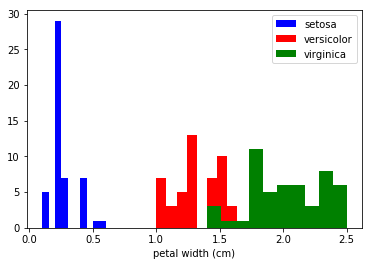### Exercise

Look at the histograms of the other features, i.e. petal length, sepal widt and sepal length.

import matplotlib.pyplot as plt
fig, ax = plt.subplots()

x_index = 3
y_index = 0

colors = ['blue', 'red', 'green']

for label, color in zip(range(len(iris.target_names)), colors):
ax.scatter(iris.data[iris.target==label, x_index],
iris.data[iris.target==label, y_index],
label=iris.target_names[label],
c=color)

ax.set_xlabel(iris.feature_names[x_index])
ax.set_ylabel(iris.feature_names[y_index])
ax.legend(loc='upper left')
plt.show()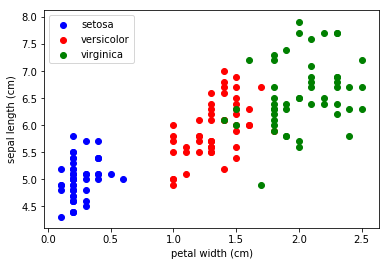### Exercise

Change x_index and y_index in the above script

Change x_index and y_index in the above script and find a combination of two parameters which maximally separate the three classes.

### Generalization

We will now look at all feature combinations in one combined diagram:

import matplotlib.pyplot as plt

n = len(iris.feature_names)
fig, ax = plt.subplots(n, n, figsize=(16, 16))

colors = ['blue', 'red', 'green']

for x in range(n):
for y in range(n):
xname = iris.feature_names[x]
yname = iris.feature_names[y]
for color_ind in range(len(iris.target_names)):
ax[x, y].scatter(iris.data[iris.target==color_ind, x],
iris.data[iris.target==color_ind, y],
label=iris.target_names[color_ind],
c=colors[color_ind])

ax[x, y].set_xlabel(xname)
ax[x, y].set_ylabel(yname)
ax[x, y].legend(loc='upper left')

plt.show()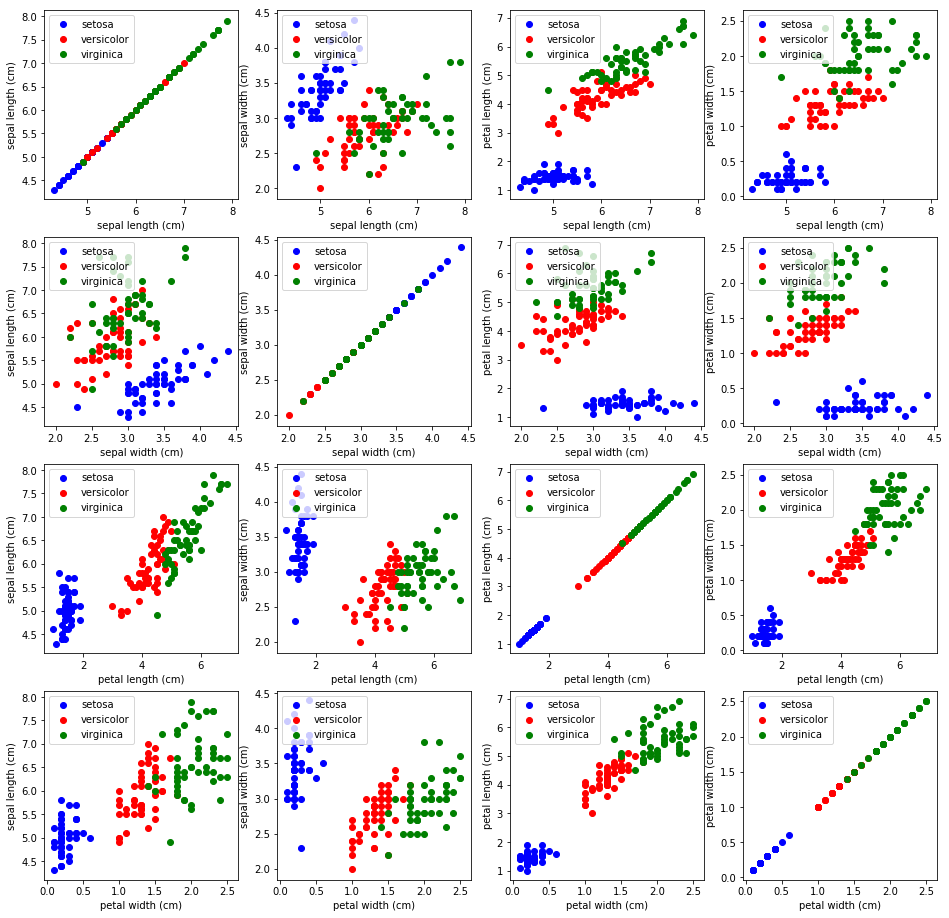## Scatterplot 'Matrices

Instead of doing it manually we can also use the scatterplot matrix provided by the pandas module.

Scatterplot matrices show scatter plots between all features in the data set, as well as histograms to show the distribution of each feature.

import pandas as pd

iris_df = pd.DataFrame(iris.data, columns=iris.feature_names)
pd.plotting.scatter_matrix(iris_df,
c=iris.target,
figsize=(8, 8)
);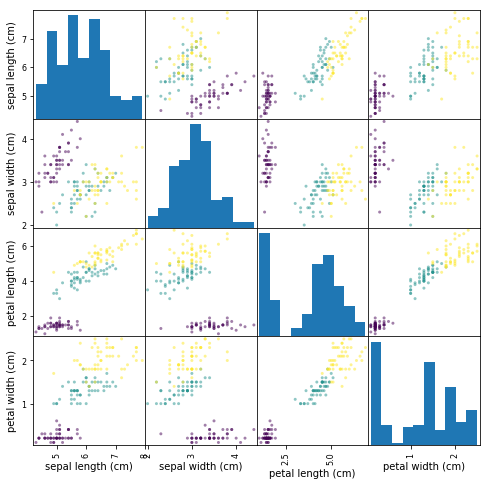## Other Available Data

Scikit-learn makes available a host of datasets for testing learning algorithms. They come in three flavors:

• Packaged Data: these small datasets are packaged with the scikit-learn installation, and can be downloaded using the tools in sklearn.datasets.load_*
• Downloadable Data: these larger datasets are available for download, and scikit-learn includes tools which streamline this process. These tools can be found in sklearn.datasets.fetch_*
• Generated Data: there are several datasets which are generated from models based on a random seed. These are available in the sklearn.datasets.make_*

You can explore the available dataset loaders, fetchers, and generators using IPython's tab-completion functionality. After importing the datasets submodule from sklearn, type

datasets.load_<TAB>



or

datasets.fetch_<TAB>



or

datasets.make_<TAB>



to see a list of available functions.

from sklearn import datasets


Be warned: many of these datasets are quite large, and can take a long time to download!

If you start a download within the IPython notebook and you want to kill it, you can use ipython's "kernel interrupt" feature, available in the menu or using the shortcut Ctrl-m i.

You can press Ctrl-m h for a list of all ipython keyboard shortcuts.

### Exercise

sklearn contains a „wine data set“.

• Find and load this data set
• Can you find a description?
• What are the names of the classes?
• What are the features?
• Where is the data and the labeled data?

### Solution

from sklearn import datasets

#print(wine.DESCR)
print(wine.target_names)
print(wine.feature_names)

data = wine.data
labelled_data = wine.target

['class_0' 'class_1' 'class_2']
['alcohol', 'malic_acid', 'ash', 'alcalinity_of_ash', 'magnesium', 'total_phenols', 'flavanoids', 'nonflavanoid_phenols', 'proanthocyanins', 'color_intensity', 'hue', 'od280/od315_of_diluted_wines', 'proline']


### Exercise:

Create a scatter plot of the features ash and color_intensity

### Solution:

from sklearn import datasets
import matplotlib.pyplot as plt

features = 'ash', 'color_intensity'
features_index = [wine.feature_names.index(features),
wine.feature_names.index(features)]

colors = ['blue', 'red', 'green']

for label, color in zip(range(len(wine.target_names)), colors):
plt.scatter(wine.data[wine.target==label, features_index],
wine.data[wine.target==label, features_index],
label=wine.target_names[label],
c=color)

plt.xlabel(features)
plt.ylabel(features)
plt.legend(loc='upper left')
plt.show()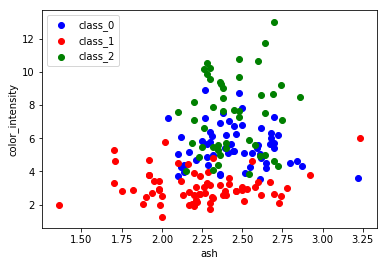### Exercise:

Create a scatter matrix of the features of the wine dataset.

### Solution:

import pandas as pd
from sklearn import datasets

def rotate_labels(df, axes):
""" changing the rotation of the label output,
y labels horizontal and x labels vertical """
n = len(df.columns)
for x in range(n):
for y in range(n):
# to get the axis of subplots
ax = axs[x, y]
# to make x axis name vertical
ax.xaxis.label.set_rotation(90)
# to make y axis name horizontal
ax.yaxis.label.set_rotation(0)
# to make sure y axis names are outside the plot area

wine_df = pd.DataFrame(wine.data, columns=wine.feature_names)
axs = pd.plotting.scatter_matrix(wine_df,
c=wine.target,
figsize=(8, 8),
);

rotate_labels(wine_df, axs)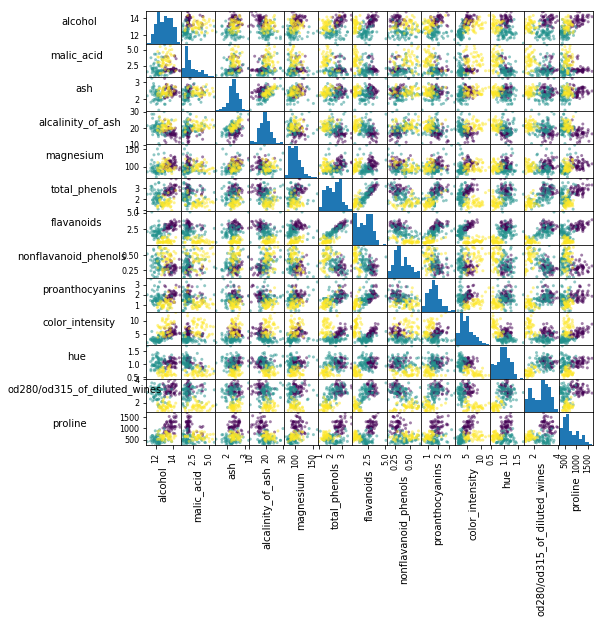Now we'll take a look at another dataset, one where we have to put a bit more thought into how to represent the data. We can explore the data in a similar manner as above:

from sklearn.datasets import load_digits

digits.keys()

Output: :
dict_keys(['data', 'target', 'target_names', 'images', 'DESCR'])
n_samples, n_features = digits.data.shape
print((n_samples, n_features))

(1797, 64)

print(digits.data)
print(digits.target)

[ 0.  0.  5. 13.  9.  1.  0.  0.  0.  0. 13. 15. 10. 15.  5.  0.  0.  3.
15.  2.  0. 11.  8.  0.  0.  4. 12.  0.  0.  8.  8.  0.  0.  5.  8.  0.
0.  9.  8.  0.  0.  4. 11.  0.  1. 12.  7.  0.  0.  2. 14.  5. 10. 12.
0.  0.  0.  0.  6. 13. 10.  0.  0.  0.]
[0 1 2 ... 8 9 8]


The target here is just the digit represented by the data. The data is an array of length 64... but what does this data mean?

There's a clue in the fact that we have two versions of the data array: data and images. Let's take a look at them:

print(digits.data.shape)
print(digits.images.shape)

(1797, 64)
(1797, 8, 8)


We can see that they're related by a simple reshaping:

import numpy as np
print(np.all(digits.images.reshape((1797, 64)) == digits.data))

True


Let's visualize the data. It's little bit more involved than the simple scatter-plot we used above, but we can do it rather quickly.

# set up the figure
fig = plt.figure(figsize=(6, 6))  # figure size in inches
fig.subplots_adjust(left=0, right=1, bottom=0, top=1, hspace=0.05, wspace=0.05)

# plot the digits: each image is 8x8 pixels
for i in range(64):
ax = fig.add_subplot(8, 8, i + 1, xticks=[], yticks=[])
ax.imshow(digits.images[i], cmap=plt.cm.binary, interpolation='nearest')

# label the image with the target value
ax.text(0, 7, str(digits.target[i]))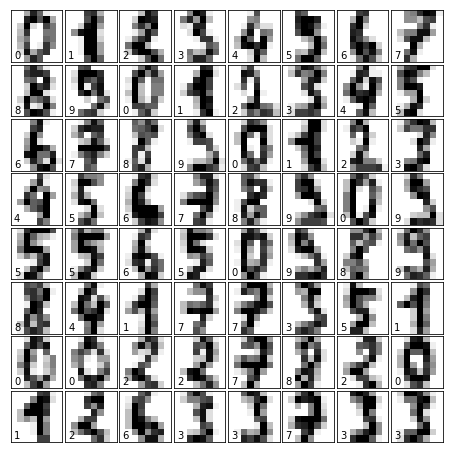We see now what the features mean. Each feature is a real-valued quantity representing the darkness of a pixel in an 8x8 image of a hand-written digit.

Even though each sample has data that is inherently two-dimensional, the data matrix flattens this 2D data into a single vector, which can be contained in one row of the data matrix.

## Exercise 02: working with the faces dataset:

Fetch the Olivetti faces dataset and visualize the faces.

from sklearn.datasets import fetch_olivetti_faces

# fetch the faces data
faces = fetch_olivetti_faces()

# Use a script like above to plot the faces image data.
# hint: plt.cm.bone is a good colormap for this data
faces.keys()

Output: :
dict_keys(['data', 'images', 'target', 'DESCR'])
n_samples, n_features = faces.data.shape
print((n_samples, n_features))

(400, 4096)

np.sqrt(4096)

Output: :
64.0
faces.images.shape

Output: :
(400, 64, 64)
faces.data.shape

Output: :
(400, 4096)
print(np.all(faces.images.reshape((400, 4096)) == faces.data))

True

# set up the figure
fig = plt.figure(figsize=(6, 6))  # figure size in inches
fig.subplots_adjust(left=0, right=1, bottom=0, top=1, hspace=0.05, wspace=0.05)

# plot the digits: each image is 8x8 pixels
for i in range(64):
ax = fig.add_subplot(8, 8, i + 1, xticks=[], yticks=[])
ax.imshow(faces.images[i], cmap=plt.cm.bone, interpolation='nearest')

# label the image with the target value
ax.text(0, 7, str(faces.target[i]))